# Cans

How many cans must be put in the bottom row if we want 182 cans arrange in 13 rows above so that each subsequent row has always been one tin less? How many cans will be in the top row?

a =  20
b =  8

### Step-by-step explanation: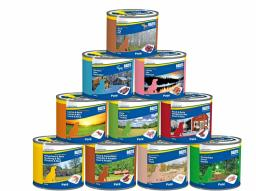Did you find an error or inaccuracy? Feel free to write us. Thank you!Tips to related online calculators
Do you have a linear equation or system of equations and looking for its solution? Or do you have a quadratic equation?

## Related math problems and questions:

• Cinema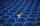How many rows of seats are in the stands, if you know that the first row has 24 seats and the last 50 seats and each subsequent row has two seats more than the row before? How many seats are in the stands?
• Chairs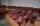Determine the number of seats in the seventh row and ninth row, if the 3rd row has 14 seats and in every next row of seats has five more than the previous row.
• Roof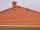Tiles are stacked in rows on the trapezoidal shaped roof. At the ridge is 15 tiles and each subsequent row has one more tile than in the previous row. How many tiled is covered roof if lowermost row has 37 tiles?
• Probably memberLook at the series 2,6,25,96,285, ? What number should come next?
• Bronze, tin and copper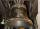Bronze is an alloy of tin and copper. An alloy of 10% tin and 90% copper is Gunmetal. If it contains 20% tin and 80% copper, it is bell metal. How many tons of molten bell metal and how many tons of copper is needed to make 100 tons of Gunmetal?
• Students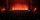In the front row sitting three students and in every other row 11 students more than the previous row. Determine how many students are in the room when the room is 9 lines, and determine how many students are in the seventh row.
• Cinema 4In the cinema are 1656 seats and in the last row are 105 seats, in each next row 3 seats less. How many are the total rows in cinema?
• Sheep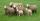Shepherd tending the sheep. Tourists asked him how much they have. The shepherd said, "there are fewer than 500. If I them lined up in 4-row 3 remain. If in 5-row 4 remain. If in 6-row 5 remain. But I can form 7-row." How many sheep have herdsman?
• Skiing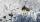Ski gondola starts at a distance of 975 m from the top and is 389 meters long. I went to the bottom station to the top station. How many meters I must go to the top of hill?
• Boxes200 boxes have been straightened in three rows. The first was 13 more than in the second, and in the second was one fifth more than in the third one. How many boxes are in each row?
• SeatsSeats in the sport hall are organized so that each subsequent row has five more seats. First has 10 seats. How many seats are: a) in the eighth row b) in the eighteenth row
• Dried fruit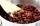The manufacturer produces a mixture of dried fruit. He purchased: 10kg pineapple for 200 Kc/kg 2kg papaya for 180 kc/kg 1kg of banana for 400 Kc/kg How many kgs of raisin for 80 Kc/kg must be put into the mix by the manufacturer so that the production pri
• Total displacementCalculate total displacement of the 4-cylinder engine with the diameter of the piston bore B = 6.6 cm and stroke S=2.4 cm of the piston. Help: the crankshaft makes one revolution while the piston moves from the top of the cylinder to the bottom and back t
• Salat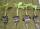Grandmother planted salad. In each row, he planted 13 seedlings. After a morning frost, many seedlings died. In the first row, five seedlings died. In the second row, two seedlings died more than 1st row. In the third row, three seedlings died less than 1
• Gp - 80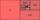One of the first four members of a geometric progression is 80. Find its if we know that the fourth member is nine times greater than the second.
• SequenceIn the arithmetic sequence is a1=-1, d=4. Which member is equal to the number 203?
• Tower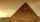Charles built a tower of cubes with an edge 2 cm long. In the lowest layer there were 6 cubes (in one row) in six rows, in each subsequent layer always 1 cube and one row less. What volume in cm3 did the whole tower have?